Create your own formulas: use the correct JavaScript syntax in conditional logic

With AODocs, you can set up conditional logic in document classes in any type of AODocs library. When users edit their documents, conditional logic is applied as follows:

• property values can be calculated automatically
• properties can be conditionally mandatory or hidden
• document titles can be calculated automatically (in Document Management libraries only)

Conditional logic is calculated using formulas that can include references to other entities such as custom or system properties, custom property states (mandatory or hidden) and the document title.

This article explains the JavaScript syntax you can use for different types of conditional logic. It also provides examples of the corresponding calculated values you can expect.

Use this article alongside the article Create your own formulas: play with entity references in JavaScript to learn how to be creative with conditional logic according to your specific business needs.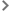Note about different types of JavaScript syntaxCalculated values for String or Text properties and calculated titlesCalculated values for Integer propertiesCalculated values for Decimal propertiesCalculated values for Boolean properties and conditionally mandatory or hidden propertiesCalculated values for Date, Date&Time or Time propertiesCalculated values for Category propertiesCalculated values for Person propertiesCalculated values for URL properties

Note about different types of JavaScript syntax

In the examples we give in our articles about conditional logic (learn more: Getting started with formulas for conditional logic), we use two types of JavaScript syntax:

• single expression
• multiple instructions, wrapped in an arrow function

You can use either in your formulas, but we recommended taking account of the following considerations.

General rule: the JavaScript code defining a formula must be made of one, and only one, expression.

This means that:

• if your logic can be expressed in a single expression, you can type it directly in the formula builder, for example:

propertyString`Project name` + ' - ' + sequenceId`document`

• if your code requires additional instructions (such as when processing dates), you should wrap all your statements in an arrow function definition (() => { ... }), and call it right after the definition with an arrow function call ():

(() => {
const d=new Date(propertyDate`Publication date`)
const yyyy=d.getFullYear()
return `\${mm}/\${dd}/\${yyyy}`
})()

Tip: To insert a multiline formula structure, start typing multi in the formula builder and select multiline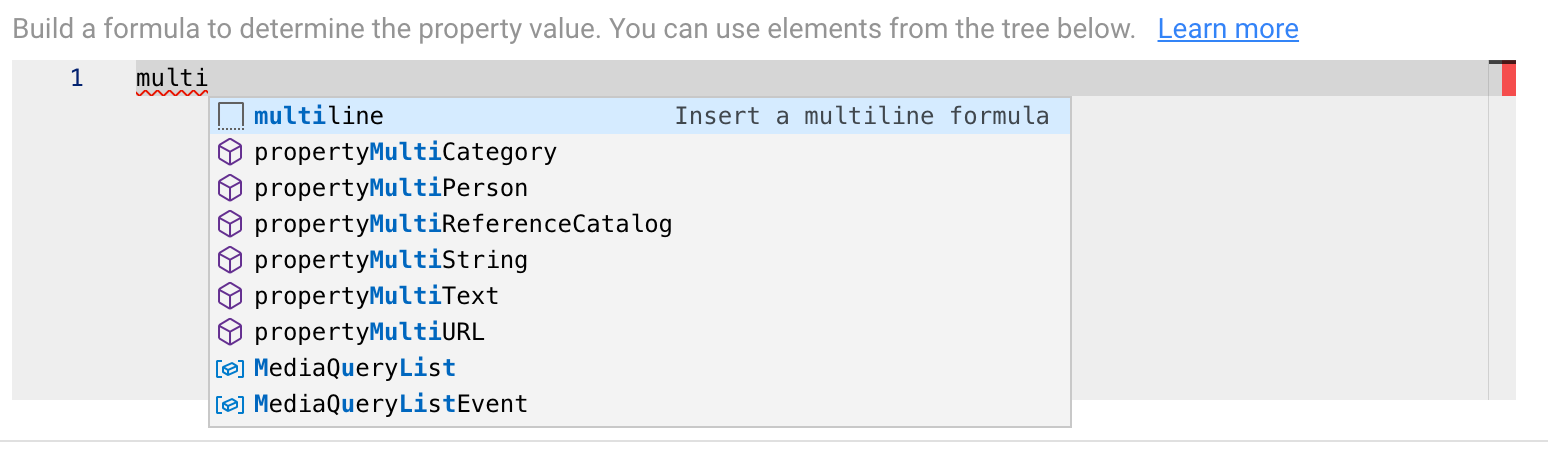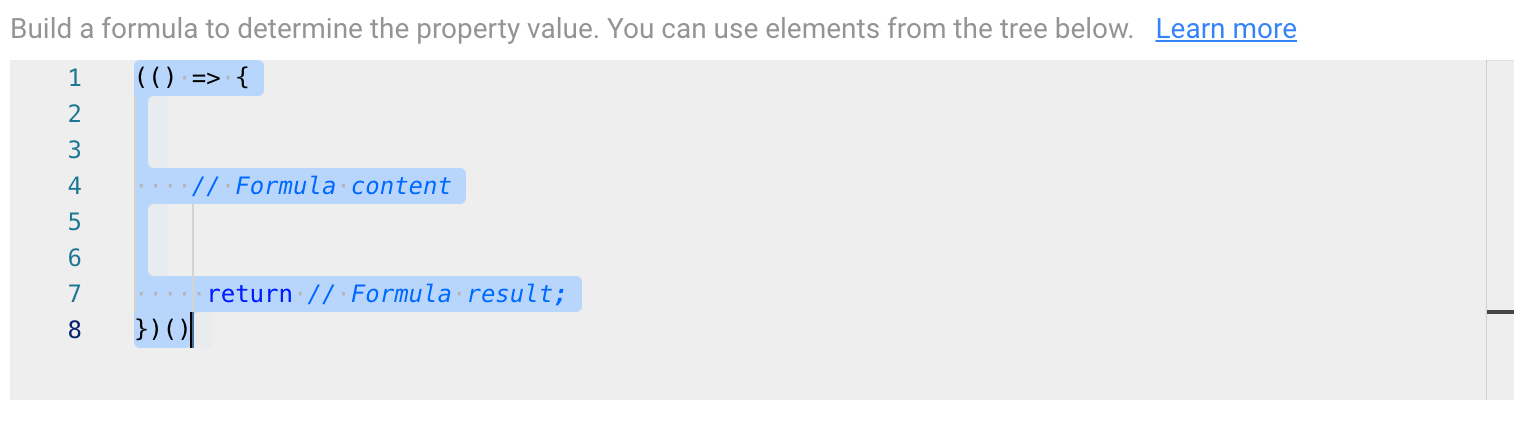However, you can play with JavaScript to combine these multiple instructions into a single expression on a single line:

`\${`\${(new Date(propertyDate`Publication date`)) .getMonth()+1}`.padStart(2,'0') }/\${`\${(new Date(propertyDate`Publication date`)) .getDate()}`.padStart( 2,'0') }/\${(new Date(propertyDate`Publication date`)).getFullYear()}`

You can then enter this directly in the formula builder without using the arrow function definition.

We recommend using the arrow function definition because with the single-line expression:

• there's a lot of repetition, which is not good practice; in our example new Date(propertyDate`Publication date`) is repeated three times
• the expression is long, which makes it difficult to read and debug

Calculated values for String or Text properties and calculated titles

String and Text properties, and also calculated titles, accept as input:

• Strings
• Integers
• Decimals
• Booleans

They also support simple math calculations, which are evaluated when the formula is calculated and the result of which is displayed. Learn more: Use simple math calculations.

Below are some supported JavaScript expressions with their expected values.

 JavaScript expression JavaScript type Calculated property value or title Comment 'Document validated' StringValue: Document validated Single quotes "Document validated" String Value: Document validated Double quotes 1234 Number Value: 1234 No formatting 3.0 Number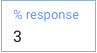Value: 3 No formatting 1+2 Number Value: 3 Evaluation first true orfalse Boolean Value: true orfalse English `\${3+4} documents` String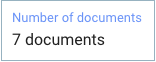Value: 7 documents String literal

Calculated values for Integer properties

The nominal expected value is a JavaScript Number, but a best effort is made to also map String types.

Below are some supported JavaScript expressions with their expected integer values.

 JavaScript expression JavaScript type Calculated property value Comment 42 Number Value: 42 Nominal integer 1+2 Number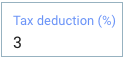Value: 3 Operation 5.0 Number Value: 5 No decimal part 3.14 Number Value: 3 Round-down 3.8 Number Value: 3 Round-down '12' String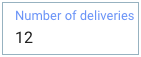Value: 12 String parsing integer "8.2" String Value: 8 String parsing decimal

Calculated values for Decimal properties

The nominal expected value is a JavaScript Number, but a best effort is made to also map String types.

Below are some supported JavaScript expressions with their expected decimal values.

 JavaScript expression JavaScript type Calculated property value Comment 3.14 Number Value: 3.14 Nominal decimal 1.2+3.8 Number Value: 5 Operation 4 Number Value: 4 Integer 2 + 3.1 Number Value: 5.1 Hybrid operation ‘6.626’ String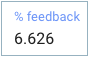Value: 6.626 String parsing decimal 1 + 0.61 Number Value: 1.61 (not 1.6099999999999999) Double precision:16 bits

Calculated values for Boolean properties and conditionally mandatory or hidden properties

For Boolean properties and conditionally mandatory or hidden properties, the nominal expected value is a JavaScript Boolean, but a best effort is made to also map String types.

Below are some supported JavaScript expressions with their expected boolean values.

 JavaScript expression JavaScript type Calculated property value or conditionally mandatory/hidden property Comment true or false BooleanValue:true or false(in this example, true) Nominal ‘true’ or ‘false’ StringValue:true or false(in this example, false) String in english ‘TRUE’ or ‘FALSE’ String Value: true or false String in ENGLISH

Calculated values for Date, Date&Time or Time properties

The nominal expected values are either JavaScript Date or Number. Strings are not supported.

Notes:
– Dates and times are given in Epoch milliseconds (the number of milliseconds since January 1, 1970, 00:00:00 UTC).
– Months in JavaScript start at zero: January is 0, February is 1, and so on. Learn more: Extract the month.
–  Date properties must use a value that corresponds to midnight UTC, otherwise an error occurs.
– The display of dates varies according to the user's locale. For example, en_GB, en_US and fr_FR all display dates differently.

Below are some supported JavaScript expressions with their expected Date values.

 JavaScript expression JavaScript type Calculated property value Comment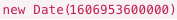Date AODocs Date property:JavaScript Date 483211100000 Number AODocs Date&Time property: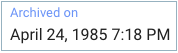Value: April 24, 1985 7:18 PM AODocs translates the number into a JavaScript Date 1607544327000 Number AODocs Time property: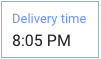Value: 8:05 PM AODocs translates the number into a JavaScript Date new Date(2015,3,1) Date AODocs Date&Time property: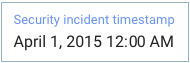Value: April 1, 2015 12:00 AM Note: If you don't indicate time in the code, the time defaults to 12 AM UTC (or 12 AM UTC plus however many hours ahead of UTC your timezone is). JavaScript Date new Date(2015,3,1,10,30) Date AODocs Date&Time property: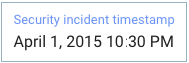Value: April 1, 2015 10:30 PM JavaScript Date

Calculated values for Category properties

The nominal expected value is a String equal to the selected category value ID

 JavaScript expression Structure of the category value entity reference:#{categoryValue:category_ID/category_value_ID} For example: '#{categoryValue:SGb0BhbtJUsWGRDo3z/SGb2pVav2f0yuuwpp9}' Important: Use the code injector in the formula builder. Learn more: What is the formula builder?. JavaScript type String Value of calculated Category property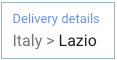Value: Italy > Lazio

Calculated values for Person properties

The nominal expected value is a String equal to the email address of the person or group you are referring to.

 JavaScript expression Email address. For example: alice@demokb.aodocs.com JavaScript type String Value of calculated Person property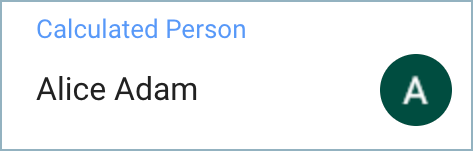Value: alice@demokb.aodocs.com

Calculated values for URL properties

The nominal expected value is a String. In its simplest form, this value is equal to the web address. It's also possible to provide a label that is displayed instead of the address. You can use the syntax: [label](address).

Below are some supported JavaScript expressions with their expected URL values.

 JavaScript expression JavaScript type Value of calculated property Comment https://www.aodocs.com String AODocs simple URL property: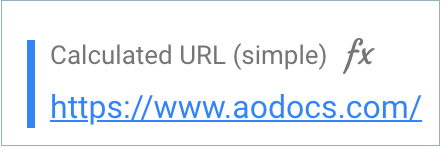The raw address is displayed in the AODocs document [AODocs website](https://www.aodocs.com) String AODocs URL property with label: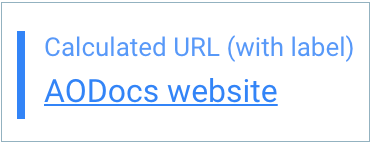The label is displayed in the AODocs document